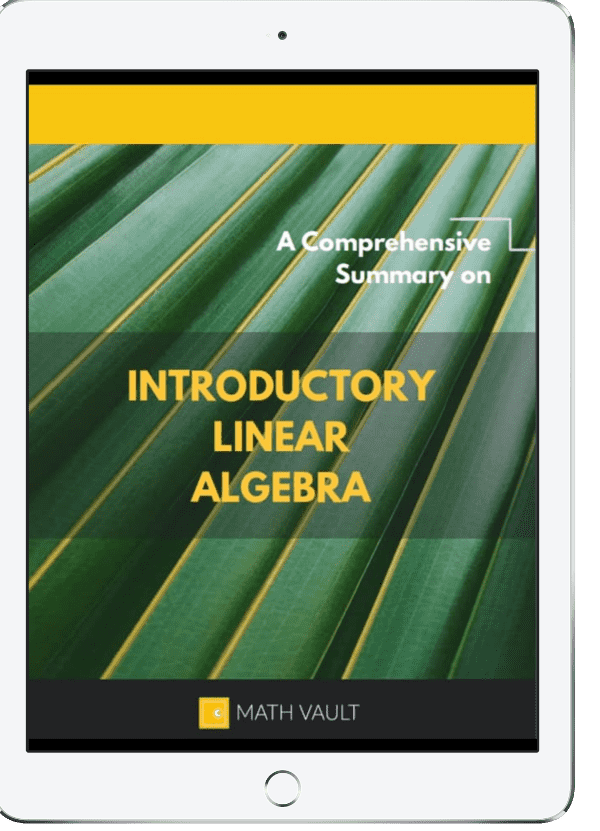## A Semester of Introductory Linear Algebra in 18 Pages?

Yes that's right. Whether you're a professor, a student or a math enthusiast, here's a guide which can help you regain your linear algebra prowess at supralinear speed.Introducing

# Comprehensive Summary on Introductory Linear Algebra

Through, comprehensive with occasional diagrams and drawings, the Comprehensive Summary serves to explore introductory linear algebra from A to Z in 14 sections:

• Linear Equations
• Matrix Operations
• Matrix Properties
• Elementary Matrices
• Invertibility
• Determinant
• Diagonalization
• Subspace
• Rank & Nullity
• Vector Geometry
• Matrix Transformation
• And more...